Quantum Chemistry / DFT
Optical / Electrical / Magnetic
Materials Science

# [Analysis Example] Birefringence and optical absorption of calcite

Targeting optical elements such as polarizers, properties of calcite were analyzed.

Calcite, a single crystal of calcium carbonate, is a well-known material that exhibits optical birefringence. Calcite, which is particularly transparent, has applications as an optical element such as polarizing plates, taking advantage of its birefringence properties. The crystal structure of calcite is hexagonal (rhombohedral) as shown in Figure 1, and the carbonate ion CO3- is carbon-centered with oxygen in an equilateral triangular arrangement (Figure 2). The reason for calcite's birefringence is the anisotropic electric polarization of the carbonate ion CO3-.In the crystal model in Figure 1, the carbonate ion CO3- is positioned parallel to the X-Y plane, but the electrical polarization response differs depending on whether the polarization direction of the electric field is parallel or perpendicular to the XY plane, resulting in birefringence.

In addition, since calcite is an insulator, its optical properties are determined by the optical transitions between the valence and conduction bands. Optical absorption, in particular, is an important clue to the electronic state because it directly reflects the band structure.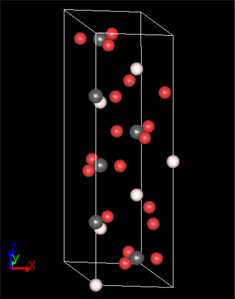Figure 1. Crystal model of calcite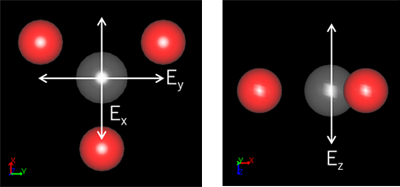Figure 2. Locations of carbon and oxygen that compose the calcium carbonate CO3- ion.
White arrows in the figure indicate the direction of polarization of the electric field.

In this case study, the optical analysis function of SIESTA was used to evaluate birefringence and band structure.

In the analysis of birefringence, the refractive index versus energy was obtained for each of the polarization directions of the electric field as (1,0,0)(0,1,0)(0,0,1) (Figure 3). For polarization directions X or Y, the refractive indices agreed within a numerical error since they are all parallel to the equilateral triangle-shaped carbonate ion CO3-. In contrast, when the polarization direction is Z, the polarization is perpendicular to the carbonate ion CO3- and the effect of electric polarization is different, resulting in different refractive indices.

Next, to evaluate the optical absorption, the extinction coefficient and energy band structure of calcite are shown in Figures 4 and 5. Figure 4 shows that optical absorption due to interband transitions begins after 6 eV. This result corresponds to the band gap in Figure 5.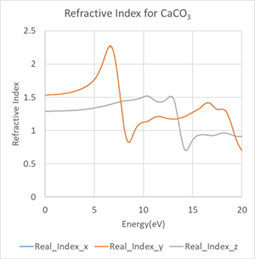Figure 3. Energy dependence of calcite refractive index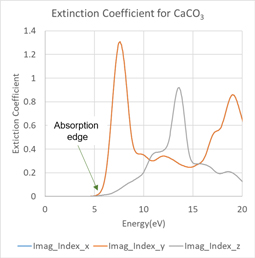Figure 4. Energy dependence of extinction coefficient of calcite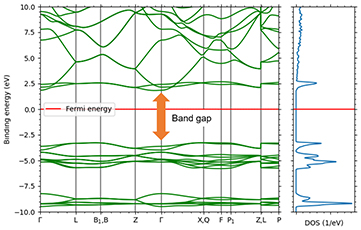Figure 5. Band structure (left) and density of states of calcite
The energy gap structure corresponding to the absorption edge in Figure 4 can be seen.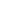This website uses cookies to improve functionality and performance. If you continue browsing the site, you are giving implied consent to the use of cookies on this website. If you want to know more or refuse consent, read our Cookie Policy.

Accept